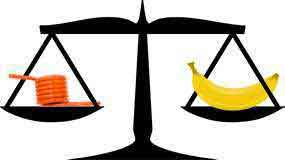<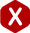# How to Calculate Spring Weight

#### Definition: The calculation of the mass weight of a coil spring based on its wire size, outer diameter, number of coils, and density of wire. Can be calculated using the corresponding formulas and equations.

Calculating the weight of your spring is necessary for both engineering and manufacturing reasons. They are required in the engineering phase when you need for this spring to be light in your application. When it comes to the making of your spring, we might need to know the weight of the spring to estimate the approximate amount of wire we will be required to use. Knowing the weight of your springs can also be very helpful when you plan on shipping them or perhaps they are being shipped to you. Using our spring calculator may help you calculate spring weight automatically but we have provided you with the formulas behind our spring calculator to help you calculate spring weight and wire length in both inches and feet.

To calculate spring weight you must calculate wire length first. The formula for wire length varies depending on the spring type. Below are the different spring calculations.

#### Calculate Wire Length

• 1.- Determine Mean Diameter (D) Add one wire diameter to the inner diameter or subtract one wire diameter from the outer diameter.
• 2.- Multiply mean diameter (D) by 3.1415 (Pi). This gives you the length of one coil in inches.
• 3.- Multiply #2 by total number of coils (N)
• 4.-This figure equals the length in inches of this spring.
##### To Determine the Total Feet in the Job
• 5.- Multiply #4 by the number of parts in the job (the quantity)
• 6.- Divide #5 by 12 to get the total feet in the job and record this number

#### Wire Length Formula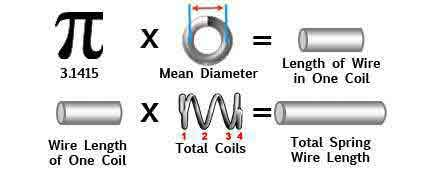#### Calculate Spring Weight

• 7.- Wire diameter x wire diameter
• 8.- #7 multiplied by 9.4245
• 9.- #8 multiplied by the density (MW / HD = 0.290, SST = 0.291, BerCu / PhBrz = 0.302)
• 10.- #9 multiplied by #6 = Total Pounds in Job
##### To Determine the Number of Feet Per 1,000 Springs
• 11.- Multiply #4 by 1,000
• 12.- Divide this figure by 12

#### Spring Weight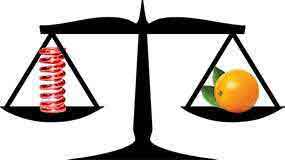#### Calculate Wire Length

• 1.- Determine Mean Diameter (D) Add one wire diameter to the inner diameter or subtract one wire diameter from the outer diameter.
• 2.- Multiply mean diameter (D) by 3.1415 (Pi). This gives you the length of one coil in inches.
• 3.- Multiply #2 by total number of coils (N) add two coils (hooks).
• 4.- This figure equals the number of inches in the spring.
##### To Determine the Total Feet in the Job
• 5.- Multiply #4 by the number of parts in the job. (the quantity)
• 6.- Divide #5 by 12 to get the total feet in the job and record this number.

#### Wire Length Formula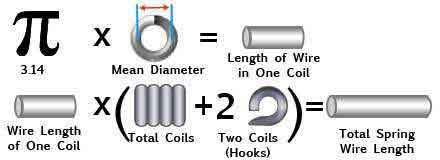#### Calculate Spring Weight

• 7.- Wire diameter x wire diameter
• 8.- #7 multiplied by 9.4245
• 9.- #8 multiplied by the density (MW / HD = 0.290, SST = 0.291, BerCu / PhBrz = 0.302)
• 10.- #9 multiplied by #6 = Total Pounds in Job
##### To Determine the Number of Feet Per 1,000 Springs
• 11.- Multiply #4 by 1,000
• 12.- Divide this figure by 12

#### Spring Weight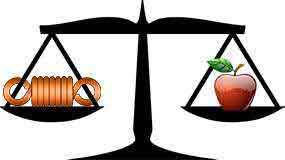#### Calculate Wire Length

• 1.- Determine Mean Diameter (D) Add one wire diameter to the inner diameter or subtract one wire diameter from the outer diameter.
• 2.- Multiply mean diameter (D) by 3.1415 (Pi). This gives you the length of one coil in inches.
• 3.- Multiply #2 by total number of coils (N)
• 4.- Add both leg lengths after doing steps #1 through #3 to finalize the calculation of inches in one spring.
##### To Determine the Total Feet in the Job
• 5.- Multiply #4 by the number of parts in the job (the quantity)
• 6.- Divide #5 by 12 to get the total feet in the job and record this number

#### Wire Length Formula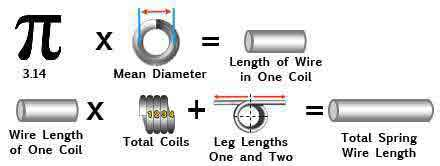#### Calculate Spring Weight

• 7.- Wire diameter x wire diameter
• 8.- #7 multiplied by 9.4245
• 9.- #8 multiplied by the density (MW / HD = 0.290, SST = 0.291, BerCu / PhBrz = 0.302)
• 10.- #9 multiplied by #6 = Total Pounds in Job
##### To Determine the Number of Feet Per 1,000 Springs
• 11.- Multiply #4 by 1,000
• 12.- Divide this figure by 12

#### Spring Weight